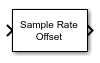# Sample Rate Offset

Apply sample rate offset to input signal

Since R2022b

•Libraries:
Communications Toolbox / RF Impairments and Components

## Description

The Sample Rate Offset block applies a sample rate offset to the input signal. Applying a sample rate offset is equivalent to changing the ADC clock rate.

## Ports

### Input

expand all

Input signal, specified as a scalar, an NS element column vector, or an NS-by-NC matrix. NS is the number of time samples. NC is the number of channels. For matrix input signals, the sample rate offset is applied independently to each column.

Data Types: `double` | `single`
Complex Number Support: Yes

### Output

expand all

Output signal, returned as a scalar, vector, or matrix. This output is the same data type as the input signal.

## Parameters

expand all

Sample rate offset in parts per million (ppm), specified as a scalar greater than –1e6.

Type of simulation to run, specified as ```Interpreted execution``` or `Code generation`.

• `Interpreted execution` — Simulate the model by using the MATLAB® interpreter. This option requires less startup time, but the speed of subsequent simulations is slower than with the ```Code generation``` option. In this mode, you can debug the source code of the block.

• `Code generation` — Simulate the model by using generated C code. The first time you run a simulation, Simulink® generates C code for the block. The model reuses the C code for subsequent simulations unless the model changes. This option requires additional startup time, but the speed of the subsequent simulations is faster than with the `Interpreted execution` option.

## Block Characteristics

 Data Types `double` | `single` Multidimensional Signals `no` Variable-Size Signals `yes`

## Version History

Introduced in R2022b